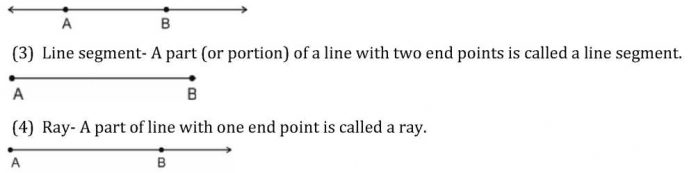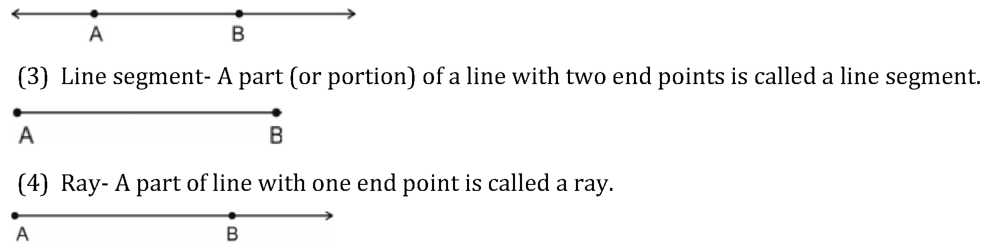# Chapter 6 Lines and Angles Notes for Class 9th Maths

0
1132Chapter – 6 Lines and Angles

1. Basic Terms and Definitions
2. Intersecting Lines and Non-Intersecting Lines
3. Pairs of Angles
4. Parallel Lines and a Transversal
5. Lines Parallel to the same Line
6. Angle Sum Property of a Triangle
7. Point- We often represent a point by a fine dot made with a fine sharpened pencil on a piece of paper.
8. Line- A line is completely known if we are given any two distinct points. Line AB is represented by as AB . A line or a straight line extends indefinitely in both the directions.(5) Collinear points- If three or more points lie on the same line, they are called collinear points otherwise they are called non-collinear points.

Types of Angles-

1. Acute angle- An acute angle measure between Oo and 90o
2. Right angle- A right angle is exactly equal to 90o
3. Obtuse angle- An angle greater than 90o but less than 180o
4. Straight angle- A straight angle is equal to 180o
5. Reflex angle- An angle which is greater than 180o but less than 360o is called a reflex angle.
6. Complementary angles- Two angles whose sum is 90o are called complementary angles.
7. Supplementary angle- Two angles whose sum is 180o are called supplementary angles.
8. Adjacent angles- Two angles are adjacent, if they have a common vertex, a common arm and their non-common arms are on different sides of common arm.
9. Linear pair- Two angles form a linear pair, if their non-common arms form a line.
10. Vertically opposite angles- Vertically opposite angles are formed when two lines intersect each other at a point.

TRANSVERSAL:

1. Corresponding angles
2. Alternate interior angles
3. Alternate exterior angles
4. Interior angles on the same side of the transversal.
• If a transversal intersects two parallel lines, then
1. each pair of corresponding angles is equal.
2. each pair of alternate interior angles is equal.
3. each pair of interior angle on the same side of the transversal is supplementary.
• If a transversal interacts two lines such that, either
1. any one pair of corresponding angles is equal, or
2. any one pair of alternate interior angles is equal or

(iii) any one pair of interior angles on the same side of the transversal is supplementary then the lines are parallel.

• Lines which are parallel to a given line are parallel to each other.
• The sum of the three angles of a triangle is 180°
• If a side of a triangle is produced, the exterior angle so formed is equal to the sum of the two interior opposite angles.